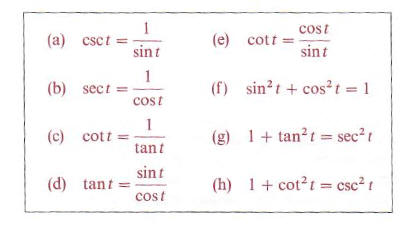Algebra

Equations

Inequalities

Graphs

Numbers

Calculus

Matrices

Tutorials

### Help: Trigonometric and hyperbolic functions

Here is a complete list of trigonometric and hyperbolic functions accepted by QuickMath. The tables show the usual form in which the functions appear in textbooks, along with the form accepted by QuickMath. In most cases, the QuickMath version is identical to the textbook version.

If there is a function missing which you would like see added to those supported by QuickMath, just send your suggestion to contact form.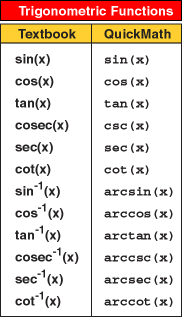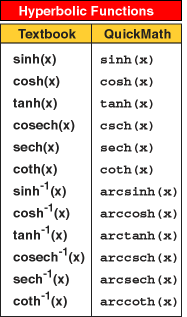# THE TRIGONOMETRIC FUNCTIONS

The wrapping function can be used to define the six trigonometric (or circular) functions. These functions are referred lo as the sine, cosine, tangent, cotangent. secant, and cosecant functions, and are designated by the symbols sin, cos, tan, cot, sec, and csc, respectively. If t is a real number, then the real number which the sine function associates with twill be denoted by either sin (t) or sin t. and similarly for the other five functions. ·

Definition of the Trigonometric Functions

If t is any real number. let P(t) be the point on the unit circle U that the wrapping function associates with t. If the rectangular coordinates of P(t) are (x.y), then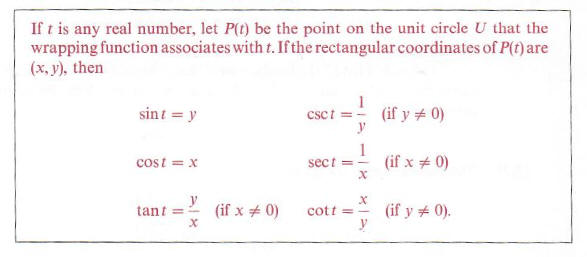If we wish to use (2.1) to find the values of the trigonometric functions corresponding to a real number t. it is necessary to determine the rectangular coordinates (x.y) of the point P(t) on U and then substitute for x and y in the definition, as illustrated in the next example. In later sections we shall introduce other techniques for finding functional values.

Example 1 Find the values of the trigonometric functions corresponding to the number t = pi/4.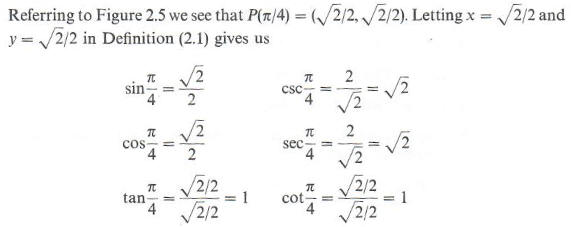Before considering additional functional values, we shall discuss several important relationships which exist among the trigonometric functions. The formulas listed below. in (2.2), are without doubt the most important identities in trigonometry, because they may be used to simplify and unify many different aspects of the subject. Since the formulas are true for every allowable value oft, and are part of the foundation for work in trigonometry, they are called the Fundamental Identities. Every student should carefully memorize (2.2) before proceeding to the next section of this text.
The last three identities in (2.2) involve squares such as (sin t)2 and (cos t)2 . In general. if n is an integer different from -1, then powers such as (cos are written in the form cosn t. The symbols sin-1 t and cos-1 t are reserved for inverse trigonometric functions to be discussed in the next chapter. With this agreement on notation we have: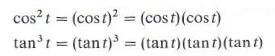and so on.

Let us first list all the fundamental identities and then discuss the proofs. The formulas to follow are true for all values of t in the domains of the indicated functions.

The fundamental identities